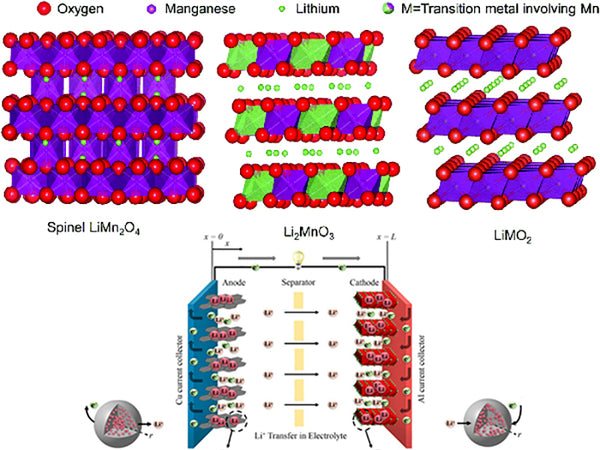• 1.Layered structure Li-Mn-O
• 2.Ion distributed
•

1. Layered structure Li-Mn-O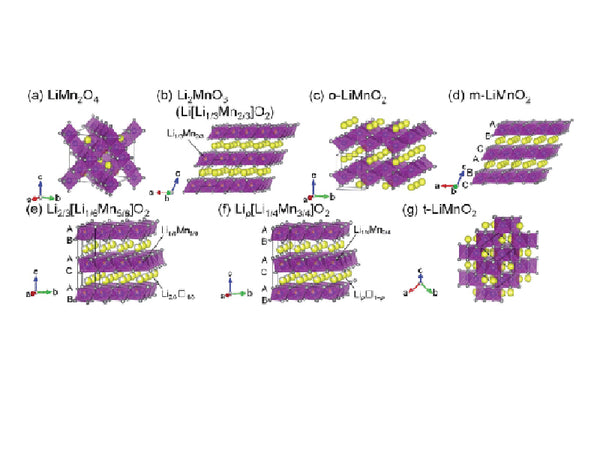Li2MnO3 is alternately filled in tightly packed oxygen planes by lithium-manganese-lithium (the ratio of lithium to manganese is 2:1). The tightly packed oxygen array deviates slightly from the ideal cubic tightly packed. The structure of Li2MnO3 is very similar to layered LiCoO2 and LiNiO2, which can be regarded as [Li]3a(Li0.33Mn0.67)3bO2, where 3a and 3b refer to the octahedral position in the triangular lattice. The arrangement of cations in Li2MnO3 reduces the symmetry of the crystal from the typical cubic symmetry of the rock salt structure to monoclinic symmetry.

There are 9 clearly distinguishable peaks on the Raman scattering spectrum of monoclinic Li2MnO3, which are located at 248cm-1, 308cm-1, 332cm-1, 369cm-1, 413cm-1, 438cm-1, 493cm-1, 568cm-1 and 612cm-1. Compared with spinel LiMn2O4, its spectral peak shifts to a lower energy direction, which is mainly caused by the difference in the chemical bonding environment between the two.

Figure 1 is an X-ray diffraction pattern of Li2MnO3 with rock salt structure and spinel compounds LiMn2O4 and Li4Mn5O12. It can be seen from this figure that the diffraction patterns of these several compounds are very similar. It's just that Li2MnO3 (135) and (2▔06) superimposed on the Bragg peak and (060) peak appear in the powder diffraction pattern with 2θ values ​​of 64.6° and 65.2° respectively, while spinel LiMn2O4 is in the 2θ range of 64°~66° There is only one (440) surface diffraction peak.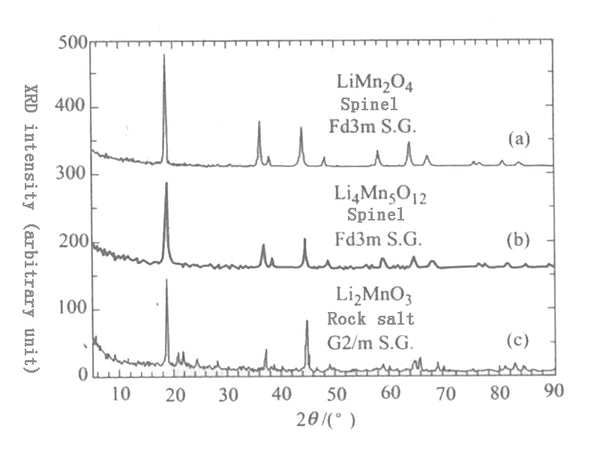Figure 1 X-ray powder diffraction patterns of several Li-Mn-O compounds
(A) Spinel LiMn2O4; (b) Spinel Li4Mn5O12;
(C) Rock salt Li2MnO3

Figure 2 shows the X-ray powder diffraction patterns of several Li-Mn-O materials such as spinel LiMn2O4, tetragonal Li2Mn2O4, monoclinic LiMnO2 and orthogonal LiMnO2. It can be seen that the patterns of monoclinic layered LiMnO2 and tetragonal spinel Li2Mn2O4 are very similar, and it is difficult to distinguish the two by X-ray diffraction alone. This is mainly due to the severe Jahn-Teller effect of Mn3+, which reduces the symmetry of spinel lithium manganese oxide, which makes lithiated spinel Li2[Mn3+]2O4 a tetragonal spinel of the I4I/amd space group structure. The cation order in the tetragonal spinel structure is very similar to that in monoclinic layered LiMnO2.Figure 2 X-ray powder diffraction pattern of several Li-Mn-O compounds. 11 (a) Spinel LiMn2O4; (b) tetragonal Li2Mn2O4; n i (c) monoclinic LiMnO2; (d) orthogonal LiMnO2

In monoclinic LiMnO2, Li+ and Mn3+ are in the 2d position in the C2/m space group, while the oxygen anion is in the 4i position. Group theory calculations show that monoclinic LiMnO2 has 3 Raman activity peaks and 6 infrared activity peaks, while the number of peaks observed in the experiment is higher than the theoretical prediction value. Three main Raman peaks of monoclinic LiMnO2 were observed, located at 419cm-1, 479cm-1 and 605cm-1, as shown in Figure 3(b). Three weak Raman peaks were detected at 575 (shoulder peak) cm-1, 358cm-1 and 286cm-1. Compared with spinel LiMn2O4, its spectral peak shifts to a lower energy direction. Therefore, it is easy to distinguish the spinel Li2Mn2O4 from monoclinic LiMnO2 with only four spectral peaks in the range of 200~650cm-1 by using Raman spectroscopy. For lithium manganese oxides with similar structures, Raman spectroscopy is an effective means to distinguish them.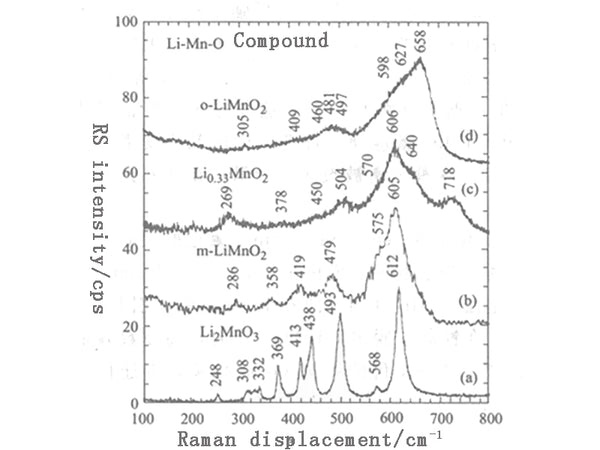Figure 3 Raman spectra of several Li-Mn-O compounds
(A) Li2MnO3; (b) monoclinic LiMnO2;
(C) Li0.33MnO2; (d) Orthogonal LiMnO2

2. Ion distributed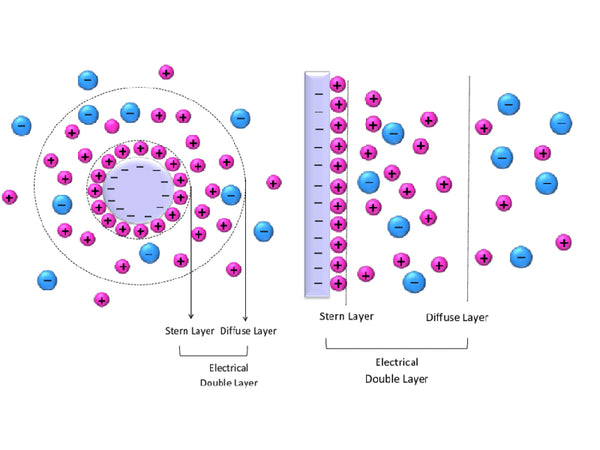The prerequisite for the design of functional materials is to first determine the microstructure of the required material, and to study the way the atoms of the material are arranged in space, that is, the distribution of ions.

1) LiCrxMn2-xO4-yAy ion distribution and capacity calculation
The battery capacity (C) refers to the amount of electricity that can be obtained from the battery under certain discharge conditions. The theoretical capacity of the battery is calculated according to Faraday's law based on the mass of the active material, that is, the mass of the substance participating in the reaction on the electrode is proportional to the amount of electricity passed. The calculation formula is as follows:
C=6.023×1023×1.6022×10-19n×(m/FW)÷3600=26.8n(m/FW)

In the formula, m is the mass of the fully reacted active material; FW is the molecular weight of the active material; n is the number of electrons gained and lost during the flow reaction. It can be seen from the above formula that when the amount of active material in the battery is determined, its theoretical capacity is inversely proportional to the molecular weight of the active material, and proportional to the number of electrons gained and lost during the flow reaction.

For the spinel lithium manganese oxide cathode material, the molecular weight FW is almost a certain value, and the number of electrons gained and lost during the flow reaction has also been fixed to 1. For the stoichiometric spinel LiMn2O4, its ion distribution can be expressed as: [Li]8a[Mn(Ⅲ)pMn(IV)q]16dO4. Li+ is located at the 8a position, Mn(Ⅲ) and Mn(IV) each account for half, located at the 16d position, and O2－ is located at the 32e position. The calculation formula of its theoretical capacity is:
C=26.8×103p/180.81(mA·h/g)

If Mn(Ⅲ) and Mn(IV) ions each account for half of the total manganese content, in this case, p=1, the theoretical capacity can be calculated to be 148.22mA·h/g. If the content of Li and Mn is low, it is possible to form metal-deficient lithium manganese oxide. The ion distribution can be expressed as: [Lix1-x]8a[Mn(Ⅲ)pMn(IV)qs]16dO4,□ Indicates holes. In this case: Li/Mn is expressed as x/(p+q)=n; electric neutrality is expressed as 3p+4q+x=8: the average valence of Mn is expressed as (3p+4q)/(p+q)=m; the total number of 16d-bit positions is expressed as p+q+s=2.

Use chemical analysis to obtain m and n, and combine the above four quaternary linear equations to obtain p. Then substitute C=26.8×103p/180.81 (mA·h/g) to calculate the theoretical capacity.

Taking the solid solution formed after Cr doping as an example, the establishment of ion distribution and the calculation of theoretical capacity are discussed. For simplicity, it is assumed that the amount of Li at the 8a position is consistent with the stoichiometric amount, and the doped Cr enters the 16d position.

First consider the cation-doped lithium manganese oxide LiCrrMn2-rO4, the ion distribution can be expressed as;
[Li]8a[Mn(Ⅲ)pMn(IV)qCr(Ⅲ)r]16dO4

The p value is calculated by the following three equations.
The total number of 16d-bit positions is expressed as: p+q+r=2
According to the principle of electrical neutrality: 3p+4q+3r=7
The average valence of Mn can be expressed as: (3p+4q)/(p+q)=m

After calculating p, substitute C=26.8×103p/180.81 (mA·h/g) to calculate the theoretical capacity.

Similarly, for the lithium manganese oxide LixCryMn2-yO4-zAz formed by doping monovalent anion A (Ⅰ) and cation Cr (Ⅲ), the ion distribution is expressed as
[Li]8a[Mn(Ⅲ)pMn(IV)qCr(Ⅲ)r]16d[O(Ⅱ)sA(Ⅰ)t]32e

The p value can be calculated by the following formulas.
According to the principle of electrical neutrality: 3p+4q+3r+1=2s+t
The total number of 16d-bit positions can be expressed as: p+q+r=2
The total number of 32e-bit positions is expressed as: s+t=4
The average valence of Mn is expressed as: (3p+4q)/(p+q)=m

After the calculated p value is substituted into the formula C=C=26.8×103p/180.81 (mA·h/g), the theoretical capacity of LixCryMn2-yO4-zAz can be calculated.

Figure 4 lists the element content and the average valence state of Mn in LixCryMn2-yO4-zAz measured by the chemical analysis method and the ion distribution of each sample calculated according to the aforementioned method.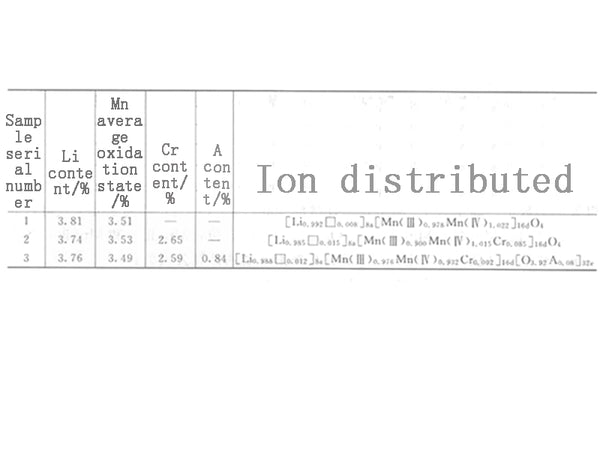Figure 4 LixCryMn2-yO4-zAz Li, Cr, A content and the average oxidation state of Mn and its ion distribution

Substituting the calculation results in Figure 4 into the formula C=26.8×103p/180.81 (mA·h/g), the theoretical capacities of sample numbers 1, 2, and 3 listed in Figure 4 can be calculated to be 144.9mA·h/g and 133.4, respectively. mA·h/g and 144.6mA·h/g.

2) Mn3O4 ion distribution and lattice constant calculation
The distribution of various cations in spinel crystals is determined by: ① ionic bond energy; ② ionic radius; ③ spatial coordination of covalent bonds; ④ influence of crystal field on the energy level and spatial distribution of d electrons. XRD, magnetic measurement, Mossbauer spectroscopy, XPS, IR and thermal analysis (ATG, DTA, DTG) and other methods can be used to study the distribution of various ions in the A and B positions and the calculation of lattice constants.

Spinel Mn3O4 has a lattice constant a=0.8363nm. In fact, this Mn3O4 is a twisted spinel type and belongs to a cubic structure. When changing from cubic to tetragonal, the relation of the lattice constant is: aT=√2aC, cT=aC. At the same time, this phase change also causes aT3% shrinkage rate and cT12% expansion rate. Based on the above relationship, the lattice constant aT=0.57356nm, cT=0.93643nm of this tetragonal spinel Mn3O4 is calculated, which is consistent with the value on the PFD card (aT=0.57621nm, cT=0.94696nm). It can be seen that as the degree of oxidation increases, the content of Mn3+ and Mn4+ at the B site in the spinel product gradually increases, and the Jahn-Teller effect becomes more obvious, that is, more and more products with a tetragonal crystal structure are formed. All transformed into tetragonal β-MnO2.